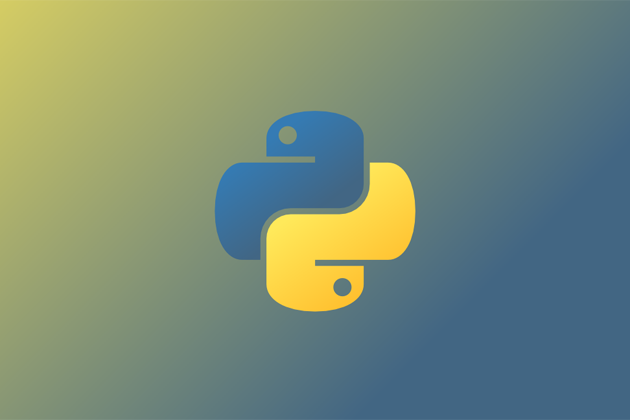# 简介FFmpeg 是一套强大的音视频处理程序，也是很多音视频软件的基础，事实上，FFmpeg 已经成为业界音视频处理的标准了。但命令行使用 FFmpeg 是有一定学习成本的，而 ffmpeg-python 库则很好的解决了这个问题。

``pip3 install ffmpeg-python``

## 获取视频信息

```probe = ffmpeg.probe(path)

video_stream = next((stream for stream in probe['streams'] if stream['codec_type'] == 'video'), None)

width = int(video_stream['width'])

height = int(video_stream['height'])```

## 镜像处理

```ffmpeg.input(path).hflip().output('output.mp4').run()

ffmpeg.input(path).vflip().output('output.mp4').run()

## 添加水印

main = ffmpeg.input(path)

logo = ffmpeg.input('logo.png')ffmpeg.filter([main, logo], 'overlay', 0, 500).output('out.mp4').run()

``ffmpeg.filter([logo, main], 'overlay', 0, 500).output('out.mp4').run()``

## 视频截取

``ffmpeg.input(path).trim(start_frame=10,end_frame=20).output('out3.mp4').run()``

## 视频拼接

`base = ffmpeg.input(path)`

base.trim(start_frame=10, end_frame=20),

base.trim(start_frame=30, end_frame=40),

base.trim(start_frame=50, end_frame=60)

output('out3.mp4').run()